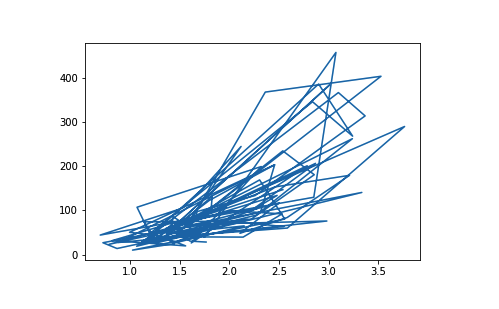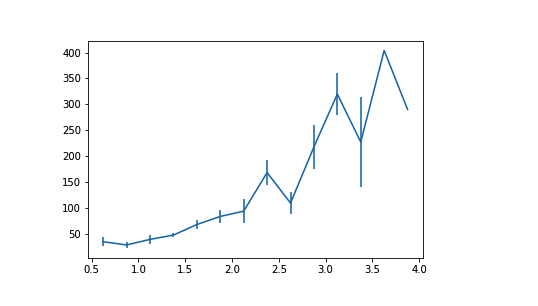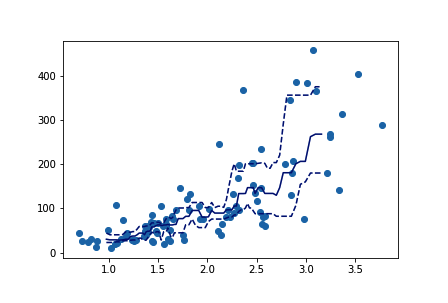# Python：matplotlib 和 Seaborn 之折线图 (三十七)

## 折线图

plt.errorbar(data = df, x = 'num_var1', y = 'num_var2')# set bin edges, compute centers
xbin_edges = np.arange(0.5, df['num_var1'].max()+0.25, 0.25)
xbin_centers = (xbin_edges + 0.25/2)[:-1]

# compute statistics in each bin
data_xbins = pd.cut(df['num_var1'], xbin_edges, right = False, include_lowest = True)
y_means = df['num_var2'].groupby(data_xbins).mean()
y_sems = df['num_var2'].groupby(data_xbins).sem()

# plot the summarized data
plt.errorbar(x = xbin_centers, y = y_means, yerr = y_sems)## 其他版本

# compute statistics in a rolling window
df_window = df.sort_values('num_var1').rolling(15)
x_winmean = df_window.mean()['num_var1']
y_median = df_window.median()['num_var2']
y_q1 = df_window.quantile(.25)['num_var2']
y_q3 = df_window.quantile(.75)['num_var2']

# plot the summarized data
base_color = sb.color_palette()
line_color = sb.color_palette('dark')
plt.scatter(data = df, x = 'num_var1', y = 'num_var2')
plt.errorbar(x = x_winmean, y = y_median, c = line_color)
plt.errorbar(x = x_winmean, y = y_q1, c = line_color, linestyle = '--')
plt.errorbar(x = x_winmean, y = y_q3, c = line_color, linestyle = '--')
plt.savefig('L4_C13_Lineplot3.png')